## prerequisites

• Use HammerDB to create TPC-H Database with Scale Factor 1 test DB.
• Create the memory optimize table

## Test

Insert 1500000 record to the disk space table takes longer than memory optimized table. Disk base table not only needs to scan the source table but also the target table.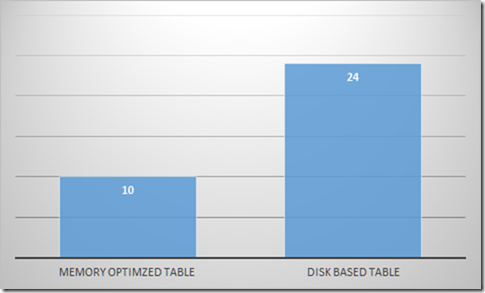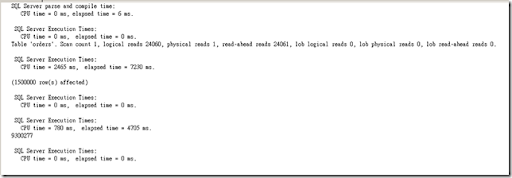LOP_HK is the new log type for in memory OLTP. HK stands for hekaton .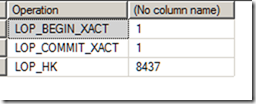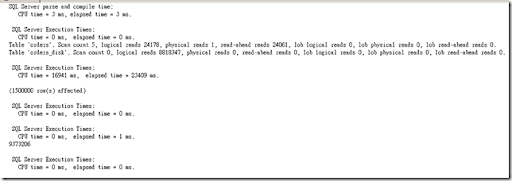LOP_LOCK_XACT means the transaction also needs to hold the lock on the table.

LOP_INSERT_ROWShas twice as the row number because the additional index we have on the table.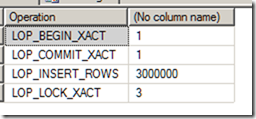When insert into the memory optimized table, we can see the memory utilization is increasing .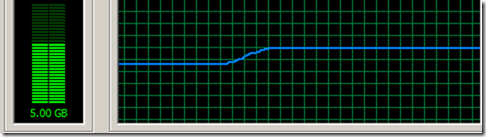`--- Compare insert operation to disk base table and memory optimized table `
` `
`drop table [orders_disk]`
`go`
`drop table [orders_mem2]`
`go`
`drop table [lineitem_mem2]`
`go`
`--- create the disk base table`
`CREATE TABLE [dbo].[orders_disk](`
`    [o_orderdate] [date] NULL,`
`    [o_orderkey] [int] NOT NULL,`
`    [o_custkey] [int] NOT NULL,`
`    [o_orderpriority] [char](15) NULL,`
`    [o_shippriority] [int] NULL,`
`    [o_clerk] [char](15) NULL,`
`    [o_orderstatus] [char](1) NULL,`
`    [o_totalprice] [money] NULL,`
`    [o_comment] [varchar](79) NULL,`
`    INDEX ix_orderkey_disk CLUSTERED (o_orderkey ASC),`
`    INDEX ix_orderdate_disk NONCLUSTERED ([o_orderdate] ASC)`
`-- CONSTRAINT [orders_pk] PRIMARY KEY NONCLUSTERED `
`) ON [PRIMARY]`
` `
`--- Create memory optimized table `
`CREATE TABLE [dbo].[orders_mem2](`
`    [o_orderdate] [date] NOT NULL,`
`    [o_orderkey] [int] NOT NULL PRIMARY KEY NONCLUSTERED HASH WITH (BUCKET_COUNT=3000000),`
`    [o_custkey] [int] NOT NULL,`
`    [o_orderpriority] [char](15) NULL,`
`    [o_shippriority] [int] NULL,`
`    [o_clerk] [char](15) NULL,`
`    [o_orderstatus] [char](1) NULL,`
`    [o_totalprice] [money] NULL,`
`    [o_comment] [varchar](79) NULL`
`    INDEX ix_orderdate (o_orderdate ASC)`
`) WITH (MEMORY_OPTIMIZED=ON, DURABILITY = SCHEMA_AND_DATA)  -- SCHEMA_ONLY`
`GO`
` `
` `
`CHECKPOINT`
`GO`
`DBCC DROPCLEANBUFFERS`
`GO`
`SET STATISTICS IO ON`
`SET STATISTICS TIME ON`
`GO`
`--- ~ about 10 secs`
`DECLARE @xact_id BIGINT`
`BEGIN TRANSACTION`
`INSERT INTO orders_mem2 SELECT * FROM orders`
`SELECT @xact_id = transaction_id FROM sys.dm_tran_current_transaction`
` `
`COMMIT`
`PRINT @xact_id`
`GO`
`--- Check tran log for insert into optimized table `
`;WITH xact_log AS`
`(`
`    SELECT `
`        [Current LSN], Operation, Context, `
`        [Transaction ID],  [Transaction Name],  [Transaction SID], [Transaction Begin], [Begin Time], [End Time],`
`        [Log Record], [Minimum LSN],`
`        [Checkpoint Begin], [Checkpoint End], Description,`
`        [Page ID],  [Dirty Pages] AS dirty_page_number,`
`        [New Split Page], [Pages Written],`
`        [Lock Information], [Number of Locks]`
`    FROM`
`        sys.fn_dblog(NULL, NULL)`
`    WHERE [Transaction ID] = (`
`            SELECT TOP (1) [Transaction ID] FROM sys.fn_dblog(NULL, NULL) WHERE [Xact ID] = 9483112`
`        )`
`)`
`SELECT Operation, COUNT(*)`
`FROM xact_log`
`GROUP BY Operation`
`-- LOP_HK    8437`
` `
`---- Run the insert on the disk base table : about 24secs`
`CHECKPOINT`
`GO`
`DBCC DROPCLEANBUFFERS`
`GO`
` `
`DECLARE @xact_id BIGINT`
`BEGIN TRANSACTION`
` `
`INSERT INTO [orders_disk]  SELECT * FROM orders`
`SELECT @xact_id = transaction_id FROM sys.dm_tran_current_transaction`
` `
`COMMIT`
`PRINT @xact_id`
`GO`
`--- Check tran log for insert into optimized table `
`;WITH xact_log AS`
`(`
`    SELECT `
`        [Current LSN], Operation, Context, `
`        [Transaction ID],  [Transaction Name],  [Transaction SID], [Transaction Begin], [Begin Time], [End Time],`
`        [Log Record], [Minimum LSN],`
`        [Checkpoint Begin], [Checkpoint End], Description,`
`        [Page ID],  [Dirty Pages] AS dirty_page_number,`
`        [New Split Page], [Pages Written],`
`        [Lock Information], [Number of Locks]`
`    FROM`
`        sys.fn_dblog(NULL, NULL)`
`    WHERE [Transaction ID] = (`
`            SELECT TOP (1) [Transaction ID] FROM sys.fn_dblog(NULL, NULL) WHERE [Xact ID] = 9483112`
`        )`
`)`
`SELECT Operation, COUNT(*)`
`FROM xact_log`
`GROUP BY Operation`
` `
` `
`--- Insert memory optimized table `
`CREATE TABLE [dbo].[lineitem_mem2](`
`    [l_shipdate] [date] NOT NULL,`
`    [l_orderkey] [int] NOT NULL,`
`    [l_discount] [money] NOT NULL,`
`    [l_extendedprice] [money] NOT NULL,`
`    [l_suppkey] [int] NOT NULL,`
`    [l_quantity] [int] NOT NULL,`
`    [l_returnflag] [char](1) NULL,`
`    [l_partkey] [int] NOT NULL,`
`    [l_linestatus] [char](1) NULL,`
`    [l_tax] [money] NOT NULL,`
`    [l_commitdate] [date] NULL,`
`    [l_receiptdate] [date] NULL,`
`    [l_shipmode] [char](10) NULL,`
`    [l_linenumber] [int] NOT NULL,`
`    [l_shipinstruct] [char](25) NOT NULL,`
`    [l_comment] [varchar](44) NULL`
`    ,PRIMARY KEY NONCLUSTERED ([l_orderkey], [l_partkey], [l_suppkey], [l_linenumber]) -- Hash Index`
`    ,INDEX ix_lineitem_mem_shipdate (l_shipdate DESC) -- Range Index`
`) WITH (MEMORY_OPTIMIZED=ON) -- Default is durable (SCHEMA_AND_DATA)`
` `
`GO`
`insert into [lineitem_mem2]  select * from lineitem`